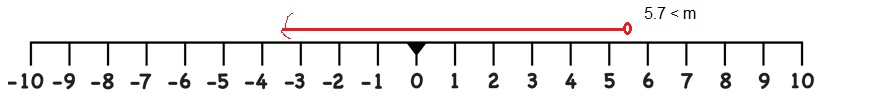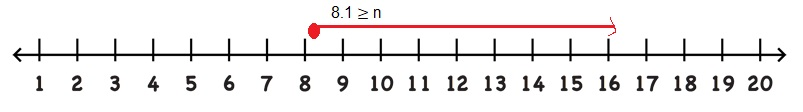# Math in Focus Grade 6 Chapter 8 Lesson 8.3 Answer Key Solving Simple Inequalities

Practice the problems of Math in Focus Grade 6 Workbook Answer Key Chapter 8 Lesson 8.3 Solving Simple Inequalities to score better marks in the exam.

## Math in Focus Grade 6 Course 1 B Chapter 8 Lesson 8.3 Answer Key Solving Simple Inequalities

### Math in Focus Grade 6 Chapter 8 Lesson 8.3 Guided Practice Answer Key

Use substitution to determine the solutions of each inequality. Then represent the solution set of each inequality on a number line.

Question 1.
h > 8
h = 8.1,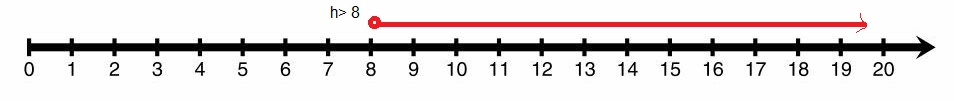Explanation:
Let us take h = 8.1 and substituting h as 8.1 we get 8.1 > 8 which is true so we can substitute h as 8.1, shown h > 8 inequality on a number line as h is greater than 8 so it starts at 8.1 and red line moves towards the right side on the number line as shown above.

Question 2.
y < 1o
y = 9.9,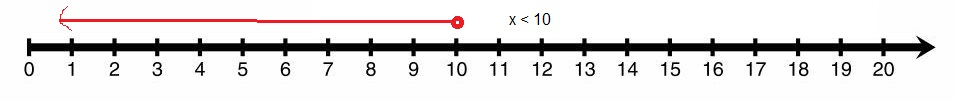Explanation:
Let us take y as 9.9 substituting y as 9.9 we get 9.9 < 10 which is true so we can substitute y as 9.9, shown y < 10 inequality on a number line as y is less than 10 so it starts at 9.9 and red line moves towards the left side on the number line as shown above.

Question 3.
p > 23
p = 23.1,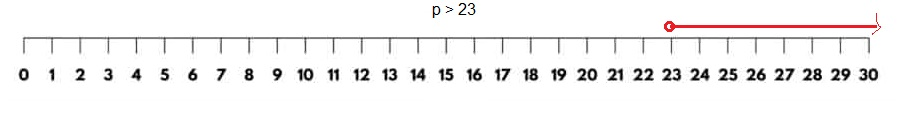Explanation:
Let us take p as 23.1 substituting p as 23.1 we get 23.1 > 23 which is true so we can substitute p as 23.1, shown p > 23 inequality on a number line as p is greater than 23 so it starts at 23.1 and red line moves towards the right side on the number line as shown above.

Question 4.
e < 14
e = 13.9,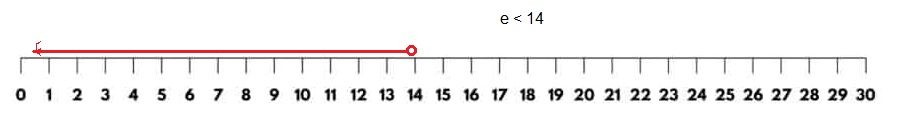Explanation:
Let us take e as 13.9 substituting e as 13.9 we get 13.9 < 14 which is true so we can substitute e as 13.9 shown e < 14 inequality on a number line as e is less than 14 so it starts at 13.9 and red line moves towards the left side on the number line as shown above.

Question 5.
m > 30
m = 30.1,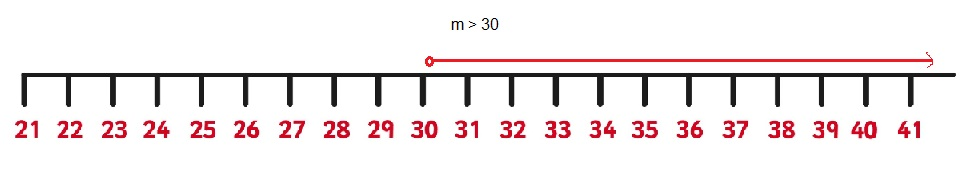Explanation:
Let us take m as 30.1 substituting m as 30.1 we get 30.1 > 30 which is true so we can substitute m as 30.1 shown m > 30 inequality on a number line as m is greater than 30 so it starts at 30.1 and red line moves towards the right side on the number line as shown above.

Question 6.
n < 5
n = 4.9,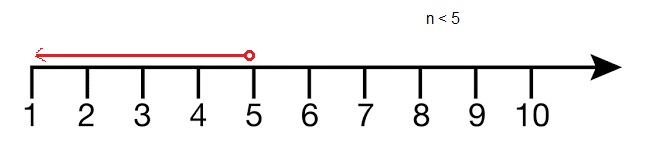Explanation:
Let us take n as 4.9 substituting n as 4.9 we get 4.9 < 5 which is true so we can substitute n as 4.9 shown n < 5 inequality on a number line as n is less than 5 so it starts at 4.9 and red line moves towards the left side on the number line as shown above.

Hands-On Activity

Writing Inequalities

Step 1.
The figure shows a balance scale.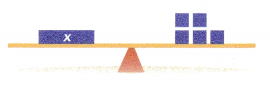Write the equation that this figure represents.

Step 2.
counters are added to the right side. Draw what the balance scale looks like now. Then write an inequality to represent the relationship between x and the counters on the right side of the balance scale.

Step 3.
3 counters are then removed from the right side. Draw what the balance scale looks like after removing the 3 counters. Then write an inequality to represent the relationship between x and the counters on the right side of the balance scale.

Step 4.
Now, if y > x, write an inequality to represent the solutions of y > x. Explain how x and y are related using a balance scale.
x = 5,

Explanation:
The equation that this figure represents is as  both are equal so x = 5,

Use substitution to find three solutions of each inequality. Then represent the solutions of each inequality on a number line.

Question 7.
q ≥ 3
q = 3, q = 4, q = 5,Explanation:
Let us take q as 3,4,5 substituting q as 3,4,5  if q = 3 then q is equal to 3 only, q = 4 means 4 is greater than 3 and q = 5 means 5 is greater than 3 we get in all 3 substitues q ≥ 3 which is true so we can substitute q as 3,4,5, shown q ≥ 3 inequality on a number line as q is greater than and equal to 3
1. It starts at 3 and red line moves towards the right side on the number line,
2. It starts at 4 and red line moves towards the right side on the number line,
3. It starts at 5 and red line moves towards the right side on the number line as shown above.

Question 8.
d ≤ 12
d = 12, d = 11, d = 10,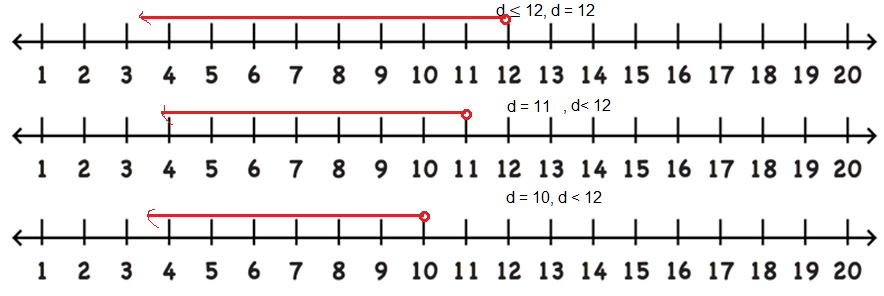Explanation:
Let us take d as 12,11,10 substituting d as 12,13,14  if d = 12 then d is equal to 12 only, d = 13 means 13 is less than 12 and q = 11 means 11 is less than 12 we get in all 3 substitues d ≤ 12 which is true so we can substitute d as 12,11,10 shown d ≤ 12 inequality on a number line as d is less than and equal to 12
1. It starts at 12 and red line moves towards the left side on the number line,
2. It starts at 11 and red line moves towards the leftt side on the number line,
3. It starts at 10 and red line moves towards the left side on the number line as shown above.

Question 9.
k ≤ 25
k = 25, k = 24, k = 23,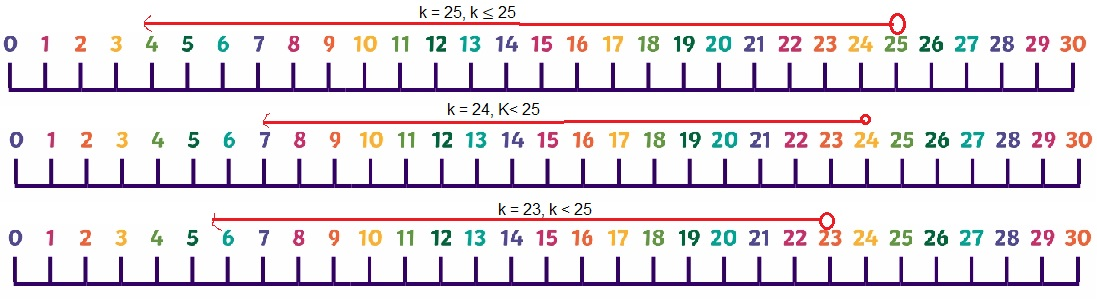Explanation:
Let us take k as 25,24,23 substituting k as 25,24,23 if k = 25 then k is equal to 25 only, d = 24 means 24 is less than 25 and d = 23 means 23 is less than 25 we get in all 3 substitues k ≤ 25 which is true so we can substitute d as 25,24,23 shown k ≤ 25 inequality on a number line as d is less than and equal to 25
1. It starts at 25 and red line moves towards the left side on the number line,
2. It starts at 24 and red line moves towards the leftt side on the number line,
3. It starts at 23 and red line moves towards the left side on the number line as shown above.

Question 10.
m ≥ -28
m = -28, m = -27, m = -26,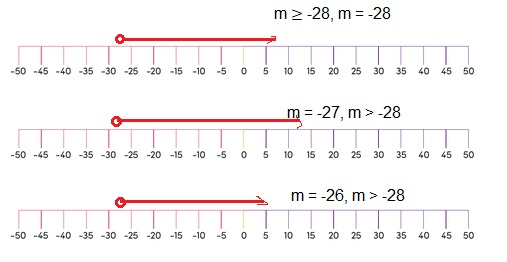Explanation:
Let us take m as -28,-27,-26 substituting m as -28,-27,-26  if m = -28 then m is equal to -28 only, m = -27 means -27 is greater than -27 and m = -26 means -26 is greater than -28 we get in all 3 substitues m ≥ -28 which is true so we can substitute m as -28,-27,-26 shown m ≥ -28 inequality on a number line as m is greater than and equal to -28
1. It starts at -28 and red line moves towards the right side on the number line,
2. It starts at -27 and red line moves towards the right side on the number line,
3. It starts at -26 and red line moves towards the right side on the number line as shown above.

Match each inequality to its graph.

a) x < 10

b) x ≤ 10

c) x > 10

d) x ≥ 10

Question 11.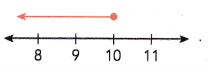b) x ≤ 10,

Explanation:
As the point on the graph starts at 10 and moves left side so it is less than and equal to 10 so it is x ≤ 10 matches with b.

Question 12.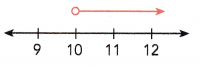c) x > 10,

Explanation:
As the point on the graphs moves from 10 towards right side it is greater than 10 so it is x > 10 matches with c.

Question 13.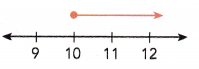d) x ≥ 10,

Explanation:
As the point on the graph starts at 10 and moves right side it is greater than and equal 10 so it is d) x ≥ 10 matches with d.

Question 14.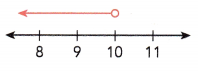a) x < 10,

Explanation:
As the point on the graphs moves from 10 towards left side it is lesser than 10 so it is x < 10 matches with a.

### Math in Focus Course 1B Practice 8.3 Answer Key

Rewrite each statement using > <, ≥, or ≤.

Question 1.
k is less than 12.
k < 12,

Explanation:
Given k is less than 12 so it is k < 12.

Question 2.
d is greater than 10.
d > 10,

Explanation:
Given d is greater than 10 so it is d > 10.

Question 3.
w is greater than or equal to 17.
w ≥ 17,

Explanation:
Given w is greater than or equal to 17 so it is w ≥ 17.

Question 4.
p is less than or equal to 36.
p ≤ 36,

Explanation:
Given p is less than or equal to 36 so it is p ≤ 36.

Question 5.
A sack of potatoes weighs at least 20 pounds. Write an inequality to represent the weight of the sack of potatoes. Answer:
x ≥ 20,

Explanation:
Given a sack of potatoes weighs at least 20 pounds. Atleast means ≥ let x be the weight of the sack of potatoes so an inequality to represent the weight of the sack of potatoes is x ≥ 20.

Question 6.
The maximum number of shirts Amanda can buy is 9. Write an inequality to represent the number of shirts that she can buy.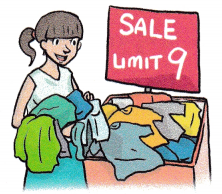x ≤ 9,

Explanation:
Given the maximum number of shirts Amanda can buy is 9. An inequality to represent the number of shirts that  she can buy is as maximum only is 9 let it be x so x ≤ 9.

Represent the solutions of each inequality on a number line.

Question 7.
x > 5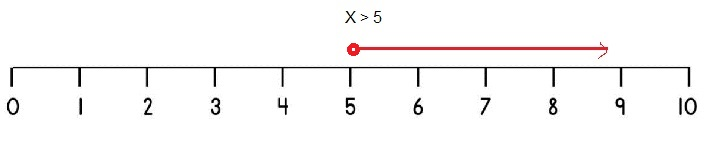Explanation:
Given to show x > 5 inequality on a number line as x is greater than 5 so it starts at 5 and red line moves towards the right side on the number line as shown above.

Question 8.
r ≥ 8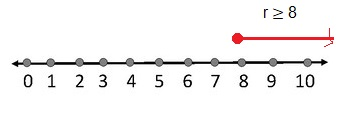Explanation:
Given to show r ≥ 8 inequality on a number line as r is greater than or equal to 8 so it starts at 8 and red line moves towards the right side on the number line as shown above.

Question 9.
m < 22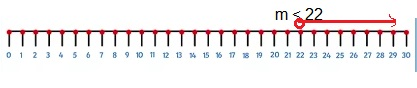Explanation:
Given to show m < 22 inequality on a number line as m is less than 22 so it starts at 22 and red line moves towards the left side on the number line as shown above.

Question 10.
q ≤ 13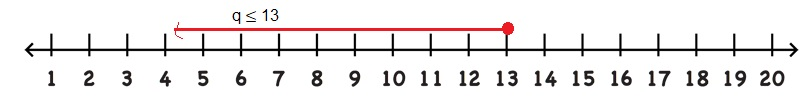Explanation:
Given to show q ≤ 13 inequality on a number line as q is less than or equal to 13 so it starts at 13 and red line moves towards the left side on the number line as shown above.

Write an inequality for each graph on a number line.

Question 11.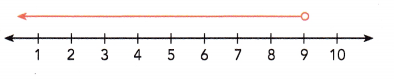x < 9,

Explanation:
Let the point be x on the graph which moves from 9 towards left side means it is lesser than 9 so the inequality is x < 9.

Question 12.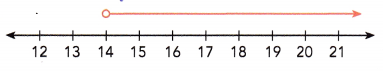x > 14,

Explanation:
Let the point be x on the graphs x moves from 14 towards right side means it is greater than 14 so the inequality is x > 14.

Question 13.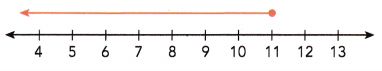x ≤ 11,

Explanation:
Let the point be x on the graph x starts at 11 and moves left side means it is less than and equal to 11 so the inequality is  x ≤ 11.

Question 14.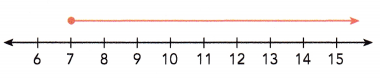x ≥ 7,

Explanation:
Let the point be x on the graph x starts at 7 and moves right side it is greater than and equal 7 means the inequality is x ≥ 7.

Represent the solutions of each inequality on a number line. Then give three possible integer solutions of each inequality.

Question 15.
p < 9$$\frac{1}{2}$$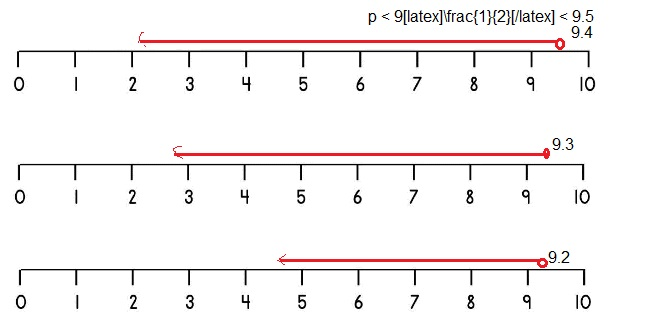Explanation:
Given p < 9$$\frac{1}{2}$$ means p < $$\frac{19}{2}$$, p < 9.5, now substituting p as 9.4,9.3,9.2 substituting p as 9.4,9.3,9.2 if p = 9.4 then 9.4 is less than 9.5, d = 9.3 means 9.3 is less than 9.5 and d = 9.2 means 9.2 is less than 9.5 we get in all 3 substitues p < 9.5 which is true so we can substitute p as 9.4,9.3,9.2 shown p < 9.5 inequality on a number line as p is less than 9.5,
1. It starts at 9.4 and red line moves towards the left side on the number line,
2. It starts at 9.3 and red line moves towards the leftt side on the number line,
3. It starts at 9.2 and red line moves towards the left side on the number line as shown above.

Question 16.
y > $$\frac{37}{5}$$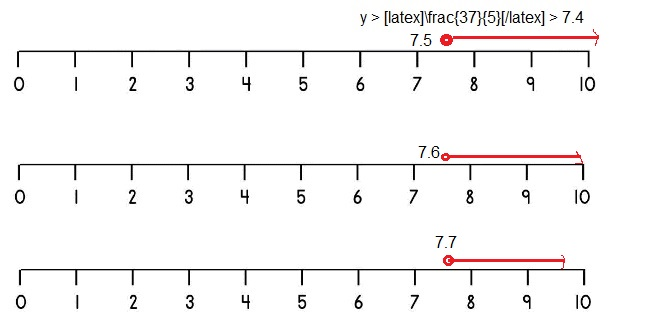Explanation:
Given y > $$\frac{37}{5}$$ means y >7.4, Let us take y as 7.5, 7.6, 7.7 substituting y as 7.5, 7.6, 7.7  if y = 7.5 then y is greater than 7.4, y = 7.6 means 7.6 is greater than 7.4 and y = 7.7 means 7.7 is greater than 7.4 we get in all 3 substitues y > 7.4 which is true so we can substitute y as 7.5,7.6,7.7, shown y > $$\frac{37}{5}$$ inequality on a number line as y is greater than to 7.4,
1. It starts at 7.5 and red line moves towards the right side on the number line,
2. It starts at 7.6 and red line moves towards the right side on the number line,
3. It starts at 7.7 and red line moves towards the right side on the number line as shown above.

Question 17.
b ≤ $$\frac{23}{4}$$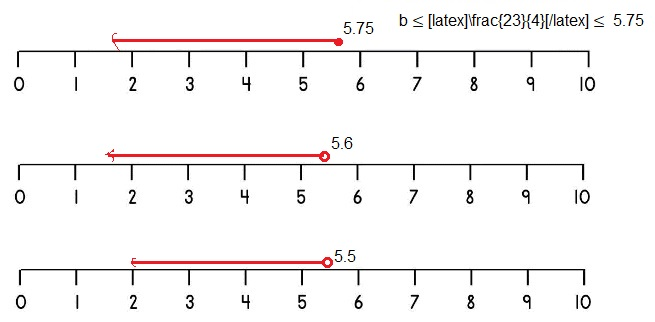Explanation:
Given b ≤ $$\frac{23}{4}$$, b ≤ 5.75, Let us take b as 5.75, 5.6, 5.5 substituting b as 5.75, 5.6, 5.5 if b = 5.75 then b is equal to 5.75 only, b = 5.6 means 5.6 is less than 5.75 and d = 5.5 means 5.5 is less than 5.75 we get in all 3 substitues b ≤ 5.75 which is true so we can substitute b as 5.75,5.6,5.5 shown b ≤ 5.75 inequality on a number line as b is less than and equal to 5.75,
1. It starts at 5.75 and red line moves towards the left side on the number line,
2. It starts at 5.6 and red line moves towards the leftt side on the number line,
3. It starts at 5.5 and red line moves towards the left side on the number line as shown above.

Question 18.
s ≥ 6$$\frac{3}{7}$$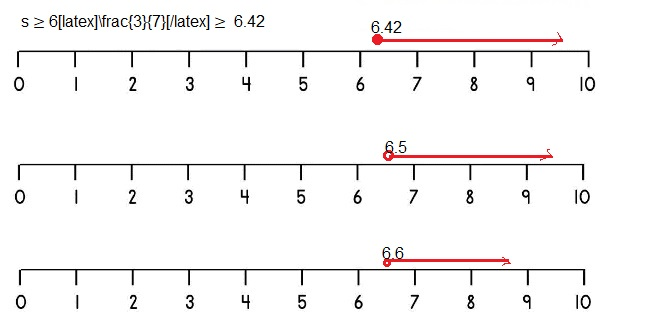Explanation:
Given s ≥ 6$$\frac{3}{7}$$ , s ≥ 6.42, Let us take s as 6.42, 6.5, 6.6 substituting s as 6.42,6.5,6.6  if s = 6.42 then s is equal to 6.42 only, s = 6.5 means 6.5 is greater than 6.42 and s = 6.6 means s is greater than 6.42 we get in all 3 substitues s ≥ 6.42 which is true so we can substitute s as 6.42,6.5,6.6 shown s ≥ 6.42 inequality on a number line as s is greater than and equal to 6.42,
1. It starts at 6.42 and red line moves towards the right side on the number line,
2. It starts at 6.5 and red line moves towards the right side on the number line,
3. It starts at 6.6 and red line moves towards the right side on the number line as shown above.

Question 19.
g > 1.5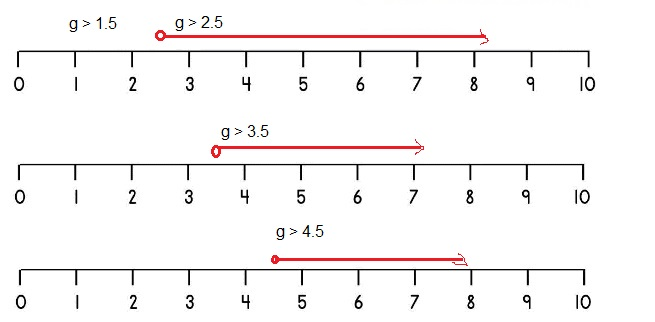Explanation:
Given g >1.5, Let us take g as 2.5, 3.5,4.5 substituting g as 2.5, 3.5, 4.5  if g = 2.5 then g is greater than 1.5, g = 3.5 means 3.5 is greater than 1.5 and g = 4.5 means 4.5 is greater than 1.5 we get in all 3 substitues g > 1.5 which is true so we can substitute y as 2.5,3.5,4.5, shown g > 1.5 inequality on a number line as g is greater than 1.5
1. It starts at 2.5 and red line moves towards the right side on the number line,
2. It starts at 3.5 and red line moves towards the right side on the number line,
3. It starts at 4.5 and red line moves towards the right side on the number line as shown above.

Question 20.
m ≥ 4.8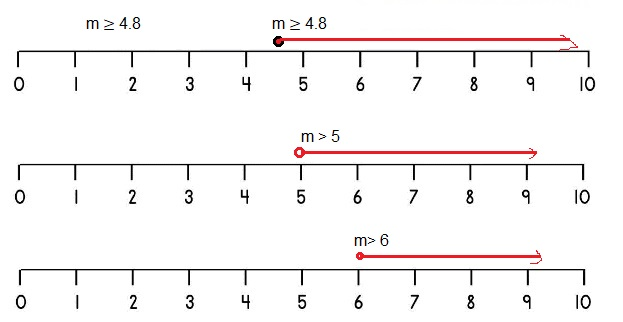Explanation:
Given m ≥ 4.8, Let us take m as 4.8, 5.0, 6.0 substituting m as 4.8,5,6 if m = 4.8 then m is equal to 4.8 only, m = 5 means 5 is greater than 4.8 and m = 6 means m is greater than 4.8 we get in all 3 substitues m ≥ 4.8 which is true so we can substitute m as 4.8,5,6 shown m ≥ 4.8 inequality on a number line as m is greater than and equal to 4.8,
1. It starts at 4.8 and red line moves towards the right side on the number line,
2. It starts at 5 and red line moves towards the right side on the number line,
3. It starts at 6 and red line moves towards the right side on the number line as shown above.

Question 21.
z ≤ 9.1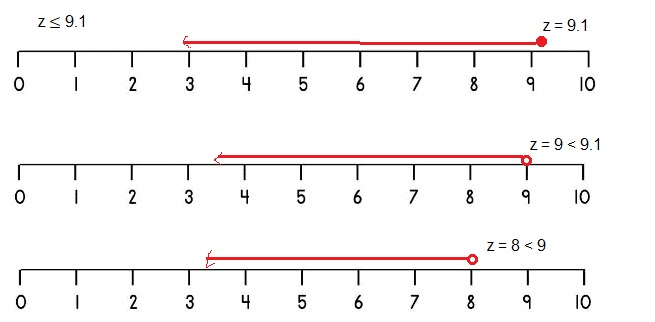Explanation:
Given z ≤ 9.1, Let us take z as 9.1, 9, 8 substituting z as 9.1,9,8 if z = 9.1 then z is equal to 9.1 only, z =9 means 9 is less than 9.1 and z = 8 means 8 is less than 9.1 we get in all 3 substitues z ≤ 9.1 which is true so we can substitute b as 9.1,9,8 shown z ≤ 9.1 inequality on a number line as b is less than and equal to ,
1. It starts at 5.75 and red line moves towards the left side on the number line,
2. It starts at 5.6 and red line moves towards the leftt side on the number line,
3. It starts at 5.5 and red line moves towards the left side on the number line as shown above.

Question 22.
r < 16.6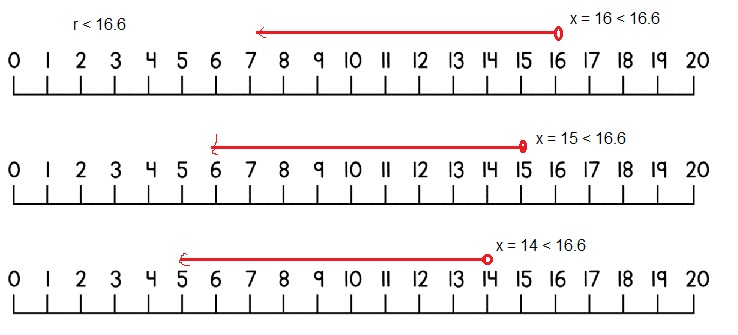Explanation:
Given r < 16.6, now substituting r as 16, 15, 14 substituting r as 16, 15 , 14 if r = 16 then 16 is less than 16.6, r = 15 means 15 is less than 16.6 and r = 14 means 14 is less than 16.6 we get in all 3 substitues r < 16.6 which is true so we can substitute r as 16,15,14 shown r < 16.6 inequality on a number line as r is less than 16.6,
1. It starts at 16 and red line moves towards the left side on the number line,
2. It starts at 15 and red line moves towards the leftt side on the number line,
3. It starts at 14 and red line moves towards the left side on the number line as shown above.

Solve.

Question 23.
In the inequality x > 9, x represents the number of restaurants along a Street.

a) Is 9 a possible value of c? Explain.
No,

Explanation:
Given the inequality x > 9, x represents the number of restaurants along a Street as x is above 9 so 9 is not possible value of c.

b) Is 9$$\frac{2}{5}$$ a possible value of x? Explain.
Yes,

Explanation:
Yes it is possible value of as given the inequality x > 9, x represents the number of restaurants along a Street as x is above 9 so 9.4 is not possible value of x.

c) Use a number line to represent the solution set of the inequality. Then state the least possible number of restaurants on the street.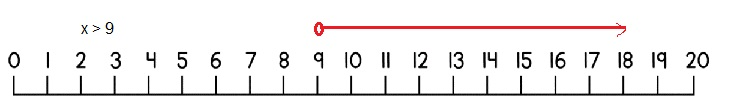The least possible number of restaurants on the street is more than 9,

Explanation:
Used a number line to represent the solution set of the inequality as shown above. Then stated the least possible number of restaurants on the street is above 9.

Question 24.
In the inequality q ≤ 24.3, q represents the possible weights, in pounds, of a package.

a) Is 24.4 a possible value of q? Explain.
No,

Explanation:
Given the inequality q ≤ 24.3, q represents the possible weights in pounds of a package. As 24.4 is not a possible value of q as q = 24.4 is not less than 24.3.

b) Is 20$$\frac{7}{10}$$ a possible value of q. Explain.
Yes,

Explanation:
Given the inequality q ≤ 24.3, q represents the possible weights in pounds of a package. As 20$$\frac{7}{10}$$ = $$\frac{207}{10}$$ =  20.7 is a possible value of q as q = 20.7 is less than 24.3.

c) Use a number line to represent the solution set of the inequality. Then state the greatest possible weight of the package.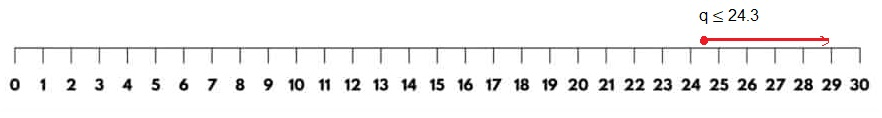Explanation:
Used a number line to represent the solution set of the inequality. The greatest possible weight of the package is 24.3.

Each inequality has the variable on the right side of the Inequality symbol. Graph each solution set on a number line.

Question 25.
11 ≤ d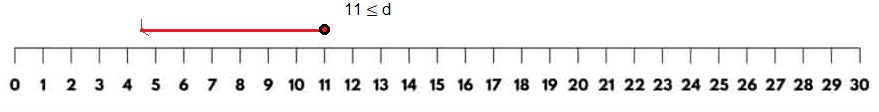Explanation:
Given inequality 11 ≤ d, Shown d is lessthan or equal to 11 on the number line above, d starts at point 11 and moves towards left side as shown above.

Question 26.
7$$\frac{3}{4}$$ > q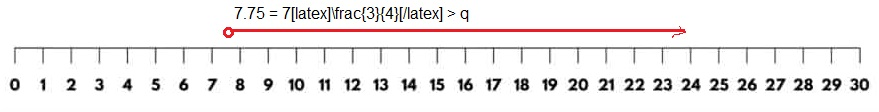Explanation:
Given inequality 7$$\frac{3}{4}$$ > q, Shown q is greater than 7$$\frac{3}{4}$$ = 7.75 on the number line above q starts above 7.75 and moves towards right side of 7.75.

Question 27.
2.5 < h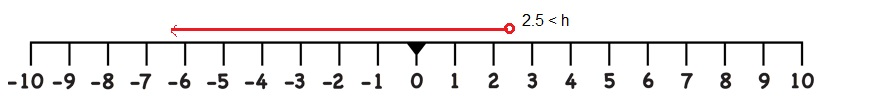Explanation:
Given inequality 2.5 < h, Shown h is lessthan to 2.5 on the number line above h starts at 2.4 and moves towards left side as shown above.

Question 28.
-6 ≥ w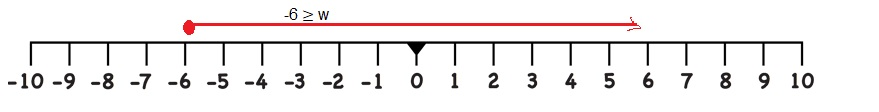Explanation:
Given inequality -6 ≥ w, Shown w is greaterthan or equal to -6 on the number line above w starts at point -6 and moves right side.

Question 29.
5.7 < m